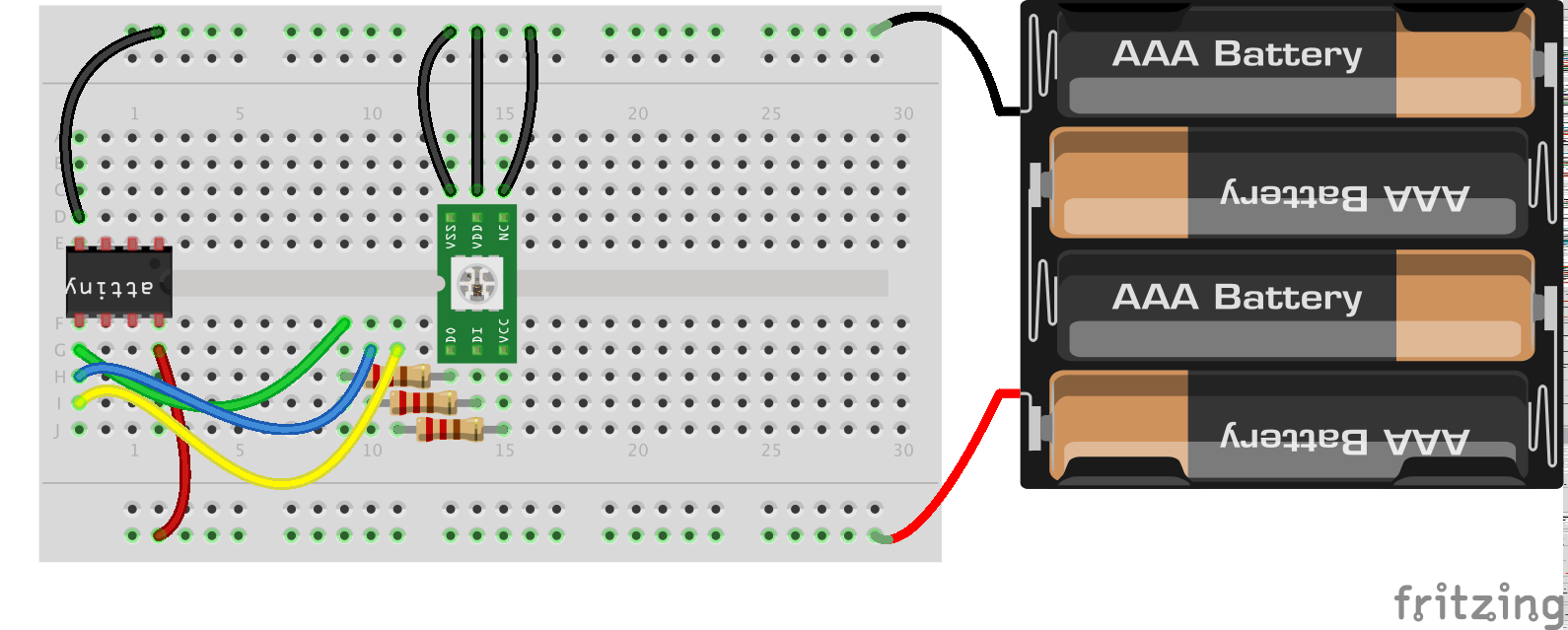### ATiny85 (Apple like) breathing light using an Arduinio

I like small electronics so when i found a sketch to make a Apple like breathing light (thanks Jeremy Saglimbeni) I decided I wanted to make it, but small. So I looked around and found a SOIC sized tiny85 chip I had laying around.

THE SKETCH

```/*
"Breathing sleep LED, like on a Mac.
Jeremy Saglimbeni 2011
thecustomgeek.com
Modified by Darren Mason 2014 bitcows.com

LED is attached to pin 11 in series with a 5.6K resistor
*/
int i = 0;
int LED_PIN = 0; //tiny85 pin 6
void setup() { // bring the LED up nicely from being off
for(i = 0 ; i <= 15; i+=1)
{
analogWrite(LED_PIN, i);
delay(5);
}
}
void loop()
{
for(i = 15 ; i <= 255; i+=1)
{
analogWrite(LED_PIN, i);
if (i > 150) {
delay(4);
}
if ((i > 125) && (i < 151)) {
delay(5);
}
if (( i > 100) && (i < 126)) {
delay(7);
}
if (( i > 75) && (i < 101)) {
delay(10);
}
if (( i > 50) && (i < 76)) {
delay(14);
}
if (( i > 25) && (i < 51)) {
delay(18);
}
if (( i > 1) && (i < 26)) {
delay(19);
}
}
for(i = 255; i >=15; i-=1)
{
analogWrite(LED_PIN, i);
if (i > 150) {
delay(4);
}
if ((i > 125) && (i < 151)) {
delay(5);
}
if (( i > 100) && (i < 126)) {
delay(7);
}
if (( i > 75) && (i < 101)) {
delay(10);
}
if (( i > 50) && (i < 76)) {
delay(14);
}
if (( i > 25) && (i < 51)) {
delay(18);
}
if (( i > 1) && (i < 26)) {
delay(19);
}
}
delay(970);
}```

THE SOFTWARE

The next we need to do is put the bootloader on this chip. To do this you will need to place the attached code in the following location. The default location on a MAC is /Users/[YOUR USER NAME]/Documents/Arduino/hardware

ARDUINO

Now lets open up the Arduino programmer app.

You need to set up your arduino first in order to program by choosing the sketch ArduinoISP: File > Examples > ArduinoISP

Make sure you have your Arduino board correctly choosen: Tools > Boards > Arduino UNO

Then make sure your programmer is set to Arduino as ISP: Tools > Programmer > Arduino as ISP